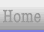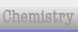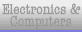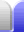# Fractions Overview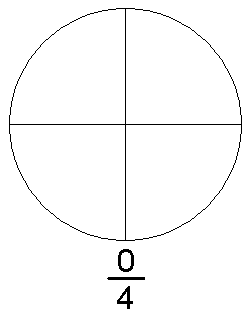Simply put, a rational number is a fraction. A rational number is one which can be made by dividing one number by another non-zero number.

The number on the top is the numerator, the number on the bottom is the denominator.

To make any number into a fraction, add a denominator of 1. It could be argued that the simplest fraction is 11.

1

=

11

13

=

131

421

=

42

 14,1281

=

14,128

Fractions can be written with a sloping line or a horizontal line. Which is used might depend on how tidy it looks, or what you are doing with it. They have exactly the same meaning.

 12=

12

1,2345,678

=

1,2345,678
• The sloping line used to write a fraction is technically known as a solidus. It's sometimes called a fraction slash. It's slightly more leaning over than a normal forward slash used in ordinary writing: ⁄ vs /.
• The horizontal line used to write a fraction is technically known as a vinculum.Home Astronomy Chemistry Electronics Mathematics Physics Field Trips Turn on javascript for email link